Courses

# Test: Some P-Block Elements Level - 1

## 25 Questions MCQ Test Chemistry Class 11 | Test: Some P-Block Elements Level - 1

Description
This mock test of Test: Some P-Block Elements Level - 1 for Class 11 helps you for every Class 11 entrance exam. This contains 25 Multiple Choice Questions for Class 11 Test: Some P-Block Elements Level - 1 (mcq) to study with solutions a complete question bank. The solved questions answers in this Test: Some P-Block Elements Level - 1 quiz give you a good mix of easy questions and tough questions. Class 11 students definitely take this Test: Some P-Block Elements Level - 1 exercise for a better result in the exam. You can find other Test: Some P-Block Elements Level - 1 extra questions, long questions & short questions for Class 11 on EduRev as well by searching above.
QUESTION: 1

### Inert pair effect of p-block elements is due to the

Solution:

Due to the presence of inner lying d and f electrons oxidation state which is 2 unit less than the group oxidation state become popular.

QUESTION: 2

### The elements of group 14 are slightly more electronegative than group 13 elements because of

Solution:

As we move along the period electronegativity increases due to increase in nuclear charge.

QUESTION: 3

### In which of the following compounds, nitrogen exhibits highest oxidation state?

Solution: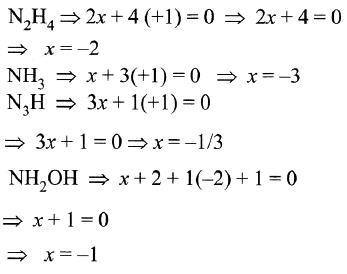QUESTION: 4

The function of Fe (OH)3 in the contact process is

Solution:

Fe (OH)3 is used to remove arsenic impurities.

QUESTION: 5

In general, nonmetals as compared to metals have

Solution:

Non metals have high value of ionization energy while metals have comparatively low value of ionization energy.

QUESTION: 6

Among the following which is the strongest oxidizing agent?

Solution:

F2 is very strong oxidizing agent.

QUESTION: 7

The compounds formed by highly reactive non-metals with highly reactive metals are generally

Solution:

Compunds formed by highly reactive non metals and metals is ionic bond.

QUESTION: 8

A compound X, of boron reacts with NH3 on heating to give another compound. Y which is called inorganic benzene. The compound X can be prepared by treating BF3 with Lithium aluminium hydride. The compounds X and Y are represented by the formulas.

Solution:

BF3 reacts with LiAlH4 to form B2H6 which reacts with NH3NH3 in 1:2 ratio forming B3N3H6( Borazine , inorganic benzene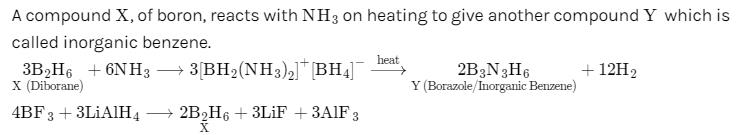QUESTION: 9

Three reactions involving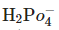are given below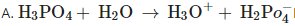B.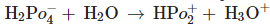C.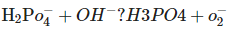In which of the above doesact as an acid?

Solution:

in B onlyis losing its proton therefore act as acid.

QUESTION: 10

When Cl2 gas reacts with hot and concentrated sodium hydroxide solution, the oxidation number of chlorine changes from

Solution:

The correct answer is option D
The reaction of chlorine gas with hot and concentrated sodium hydroxide solution is
3Cl2​ + 6NaOH⟶NaClO3​+ 5NaCl+3H2​O
Oxidation number of Cl is 0 in Cl2​, −1 in NaCl and +5 in NaClO3
So the oxidation number of chlorine changes from Zero to -1 and Zero to +5.

QUESTION: 11

As we move from B to Al in the p-block elements the sum of the first three ionisation enthalpies

Solution:

On moving from B to Al size increases so sum of ionization energies also decreases.

QUESTION: 12

The non-metal oxides are:

Solution:

nonmetallic oxides are either neutral or acidic. Example CO is neutral while CO2 is acidic.

QUESTION: 13

Dry ice is

Solution:

Dry ice is solid CO2

QUESTION: 14

The tendency of BF3, BCl3 and BBr3 to behave as Lewis acid decreases in the sequence:

Solution:

because in BF3 there is backbonding which decreases its acidity. And as we for from BF3 to BBr3 tendency of back bonding decreases so acidity increases,.

QUESTION: 15

Of the following compounds the most acidic is

Solution:

More is the oxidation number greater is the acidity of oxide

QUESTION: 16

Boron forms only covalent compounds and not +3 ions because

Solution:

Due to smaller size and high nuclear charge B has very high ionization energy value so doesnot show +3 oxidation state

QUESTION: 17

The element having the noble gas plus 14 f- electrons plus 10 d-electron cores

Solution:

Tl have bole gas + 14 f +10 d electrons.

QUESTION: 18

In trivalent state, for example, the trichlorides, being covalent are hydrolysed in water form

Solution:

trihalides hydrolysis to form tetrahedral structure

QUESTION: 19

Which of the following statements regarding ozone is not correct?

Solution:

bond order of O-O bond in ozone is less than that in oxygen.

QUESTION: 20

XeF2 is isostructural with

Solution:

The correct answer is option D

XeF2 is isostructural with ICl2−.
Both contain the same number of lone pairs and bond pairs on the central atom.
There are 3 lone pairs and 2 bond pairs.
This results in linear geometry.

QUESTION: 21

Aqueous solution of Na2S2O3 on reaction with Cl2 gives

Solution:

Aqueous Na2S2O3 on reaction with Cl2Cl2 produces NaHSO4

QUESTION: 22

Which noble gas is most abundant in atmosphere?

Solution:

Argon

Argon is most abundant in composition of air percent by volume. It is in 0.934% in composition of air and is one of the major gas in the Earth's atmosphere.

QUESTION: 23

Aqueous solution of Na2S2O3 on reaction with Cl2Cl2 gives

Solution:

Aqueous Na2S2O3 on reaction with Cl2Cl2 produces NaHSO4

QUESTION: 24

One of the following p-block elements has unusually low melting point

Solution:

Ga has very low melting point.

QUESTION: 25

Which of the following statements regarding ozone is not correct?

Solution:

bond order of O-O bond in ozone is less than that in oxygen.